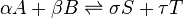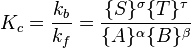# Equilibrium facts for kids

Kids Encyclopedia Facts

An equilibrium is a state of a system where all forces acting on the system is balanced. A system that is in equilibrium does not change. The word has been used for different concepts from different fields of study.

• Hydrostatic equilibrium applies to liquids.
• Thermal equilibrium means as much heat is entering and leaving something.
• Homeostasis is a living thing keeping its internal balance.

## Chemical equilibrium

Chemical equilibrium is an idea which describes the behavior of chemical reactions over time. In reactions that have finished reacting and have attained equlibrium, the concentrations of the reactant and product chemicals do not change. An example of an equilibrium reaction is as follows:$\alpha A + \beta B \rightleftharpoons \sigma S + \tau T$

In the example above, the double arrow shows that the reaction is an equilibrium reaction. This means that the forward reaction and the backward reaction can happen, usually due to a small enthalpy change in each reaction. Hence, the amount of product chemicals to reactant chemicals remains a fixed value, and such a ratio is called the equilibrium constant (Kc).

### Equilibrium constant

The equilibrium constant is a ratio of the concentration of the products raised to the appropriate powers to the concentration of the reactants raised to the appropriate powers. In the above example, the equilibrium constant is as follows:$K_c=\frac{k_b}{k_f}=\frac{\{S\}^\sigma \{T\}^\tau } {\{A\}^\alpha \{B\}^\beta}$

For some reactions, the equilibrium constant is very large, in which case nearly all of the reactant chemical is turned into products, such as when paper is burned. For other reactions, it is very small, which results in very little products being formed. If the equilibrium constant is close to 1, a mixture of both products and reactants is expected at equilibrium.

When chemists talk about equilibrium constants, they call reactions with large K values favorable and reactions with small K values unfavorable. It is important to note, however, these terms are not judgements about the value or usefulness of a particular chemical reaction. In fact, some very important reactions are actually "unfavorable"; one such example is the synthesis of ammonia from nitrogen and hydrogen gas (Haber process), which has a rather small equilibrium constant.

Understanding how chemical equilibriums work is important as it helps in understanding how a reaction happens. It allows chemists to calculate how much products will be formed from a reaction.

### Le Chatelier's principle

The concept of Le Chatelier's principle is important in predicting how an equilibrium will change when different factors are changed in a system. For example, using the Le Chatelier's principle, we are able to predict how the position of equilibrium will change when the concentration of a certain reactant/product has changed, as the system will try to counteract the change by favoring either the forward or the backward reaction.Equilibrium Facts for Kids. Kiddle Encyclopedia.